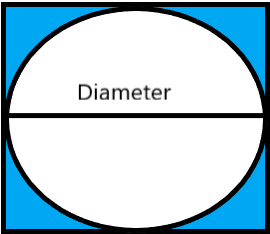QuestionAnswers

# The density of mercury is $13.6g/ml$. The approximate diameter of an atom of mercury assuming that each atom is occupying a cube of edge length equal to the diameter of mercury atom is $a=2.9004\times {{10}^{-x}}cm$. The value of x is _____. (Given atomic mass of mercury is $200.59u$)Hint: The structure is cubic and we have to find out its volume ($V=\dfrac{4}{3}\pi {{r}^{3}}$). Then we have to apply the density formula to find the unknown variable ($Density=\dfrac{Mass}{Volume}$ ).

Complete step by step solution:
Given data:
The density of mercury (D) is given as $13.6g/ml$. The diameter of a mercury atom is$a=2.9004\times {{10}^{-x}}cm$which is also equal to the edge length of the cube inside which this atom is placed. The shape is somewhat like this:We also know the mass of a mercury atom. It is $200.59u$ where “u” is the atomic mass unit. Converting it into grams it becomes
$200.59u\times 1.66\times {{10}^{-24}}=3.329\times {{10}^{-22}}g$
The volume (V) of atom is $\dfrac{4}{3}\pi {{r}^{3}}$
\begin{align} & \Rightarrow V=\dfrac{4}{3}\times 3.14\times {{(1.4502\times {{10}^{-X}})}^{3}} \\ & \Rightarrow V=12.768\times {{10}^{-3X}}c{{m}^{3}} \\ \end{align}
Now we have the values of all the variables required for this question. Below we put them together systematically and try to solve them. We start with the formula for density:
\begin{align} & Density(D)=\dfrac{Mass(M)}{Volume(V)}=\dfrac{3.329\times {{10}^{-22}}g}{12.768\times {{10}^{-3x}}c{{m}^{3}}} \\ & D=0.26\times {{10}^{-22+3x}}g/c{{m}^{3}} \\ & \text{As we know density is given as D=13}\text{.6g/ml or 13}\text{.6c}{{\text{m}}^{3}} \\ & \text{So the above equation becomes:} \\ & \Rightarrow 13.6=0.26\times {{10}^{-22+3x}} \\ & \Rightarrow {{10}^{-22+3x}}=\dfrac{13.6}{0.26}=52.3 \\ & \text{Taking the log of both sides we get:} \\ & \Rightarrow (3x-22)log(10)=log(52.3) \\ & \Rightarrow 3x-22=1.718 \\ & \Rightarrow 3x=23.718 \\ & \Rightarrow x=7.906\approx 8 \\ \end{align}
The value of the unknown x = 8

So, the diameter of the atom or the edge of the cube $a=2.9004\times {{10}^{-8}}cm$.

Note: The question gives the data that the edge length of the cube is the same as the diameter of the sphere. If here a student takes the volume of the cube rather than that of the sphere it would be wrong. You have to remember that the density given is that of mercury, which means it relates to the atoms of the element and not the space they occupy at the atomic level.
As you can see in the picture represented at the start, the volume of the cube with the given value of edge length is more than that of the sphere.

The Solid StateWhat is the full form of phd?What is the Cell Envelope?What is the Placebo EffectWhat is the Difference Between Trade and Commerce?The Idea of TimeWhat are the Functions of the Human Skeletal System?The Ghat of the Only World SummaryThe Perimeter of Rectangle FormulaDark Side of the MoonImportant Questions for CBSE Class 12 Chemistry Chapter 1 - The Solid StateImportant Questions for CBSE Class 11 Chemistry Chapter 11 - The p-Block ElementsImportant Questions for CBSE Class 11 Chemistry Chapter 10 - The s-Block ElementsNCERT Books Free Download for Class 12 Chemistry Chapter 1 - The Solid StateImportant Questions for CBSE Class 11 English Snapshots Chapter 1 - The Summer of the Beautiful White HorseNCERT Books Free Download for Class 11 Chemistry Chapter 11 - The p-Block ElementsImportant Questions for CBSE Class 11 Biology Chapter 8 - Cell The Unit of LifeCBSE Class 8 Science Reaching The Age of Adolescence WorksheetsImportant Questions for CBSE Class 11 English Hornbill Chapter 5 - The Ailing Planet: the Green Movement's RoleImportant Questions for CBSE Class 11 English Woven Chapter 6 - The StoryChemistry Question Paper for CBSE Class 12CBSE Class 12 Chemistry Question Paper 2020Chemistry Question Paper for CBSE Class 12 - 2013Chemistry Question Paper for CBSE Class 12 - 2015CBSE Class 12 Chemistry Question Paper 2019 - Free PDFCBSE Class 12 Chemistry Question Paper 2017 - Free PDFCBSE Class 12 Chemistry Question Paper 2018 - Free PDFPrevious Year Question Paper for CBSE Class 12 Chemistry - 2014Chemistry Question Paper for CBSE Class 12 - 2016 Set 1 EChemistry Question Paper for CBSE Class 12 - 2016 Set 1 SNCERT Solutions for Class 12 Chemistry Chapter 1 The Solid State in HindiNCERT Solutions Class 11 English Woven Words Prose Chapter 4 The Adventure of the Three GarridebsLakhmir Singh Chemistry Class 9 Solutions Chapter 4 - Structure Of The AtomNCERT Solutions for Class 11 Chemistry Chapter 11 The p-Block Elements In HindiNCERT Solutions for Class 12 Chemistry Chapter 1NCERT Solutions for Class 11 English Snapshots Chapter 6 - The Ghat of the Only WorldNCERT Solutions for Class 11 Chemistry Chapter 10 The S-Block Elements In HindiNCERT Solutions Class 11 English Woven Words Poem Chapter 5 The World is too Much With UsNCERT Solutions for Class 11 English Hornbill Chapter 4 - Landscape of the SoulRS Aggarwal Class 11 Solutions Chapter-16 Conditional Identities Involving the Angles of a Triangle